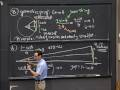Session 7: Derivatives of Sine and Cosine« Previous | Next »

Overview

The sine and cosine functions are used to describe periodic phenomena such as sound, temperature and tides. Trying to differentiate these functions leaves us with two limits to investigate further.

Lecture Video and Notes

Video Excerpts

Flash and JavaScript are required for this feature.From Lecture 3 of 18.01 Single Variable Calculus, Fall 2006

Flash and JavaScript are required for this feature.» Clip 2: Derivative of cos(x) (00:07:00)

From Lecture 3 of 18.01 Single Variable Calculus, Fall 2006

Recitation Video

Derivatives of Sine and Cosine

Flash and JavaScript are required for this feature.

Worked Example

Geometric Derivatives of Sine and Cosine

Use the mathlet below to complete the worked example.

Mathlet

Creating the Derivative

« Previous | Next »